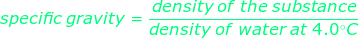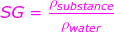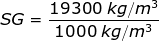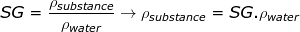# Specific Gravity Formula

Specific Gravity Formula

Specific gravity is a measure of relative density. The specific gravity is the density of a substance divided by the density of water. Density is measured in the units kg/m3. The density of water at 4.0°C is 1000 kg/m3. So, the specific gravity is a unitless number.SG = specific gravity (unitless)

ρsubstance = the density of the substance (kg/m3)

ρwater = the density of water at 4.0°C, 1000 kg/m3

Specific Gravity Formula Questions:

1) The density of gold is ρgold = 19300 kg/m3. What is the specific gravity of gold?

Answer: The specific gravity can be found by dividing the density of gold by the density of water at 4.0°C:SG = 19.3

The specific gravity of gold is 19.3.

2) A The specific gravity of ice is 0.92. What is the density of ice, and is it greater or less than the density of water at 4.0°C (liquid water)?

Answer: The density of ice can be found by rearranging the specific gravity equation:ρsubstance = 0.92∙1000 kg/m3

ρsubstance = 920 kg/m3

The density of ice is 920 kg/m3, which is less than the density of liquid water. This is a very rare property of water compared to other materials. The density of the solid form of a substance is almost always greater than the density of the liquid form. Besides water, the most "common" substance that has this property is plutonium.